Our World Statistics Day conversations have been a great reminder of how much statistics can inform our lives. Do you have an example of how statistics has made a difference in your life? Share your story with the Community!
Choose Language Hide Translation Bar

## Sample Size and Power for Testing Two Variances

This add-in will estimate either the sample size or the power for testing two variances as an F-ratio. It is designed to work like the sample size and power calculators available through the JMP DOE menu.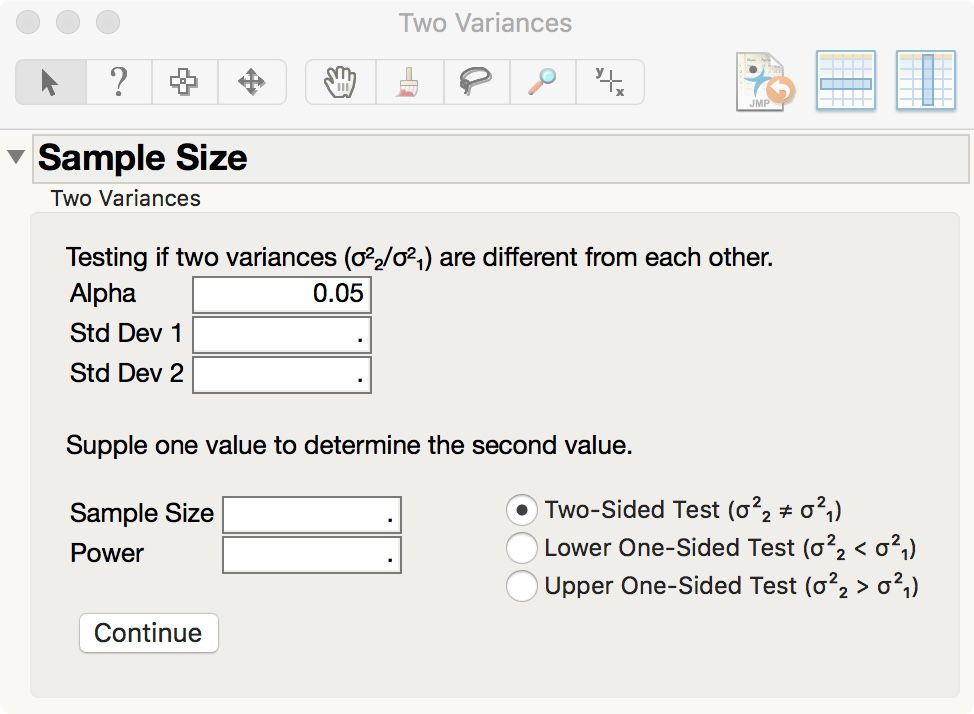The initial window includes a default significance level. Enter two standard deviations that represent the minimum difference that is important. Std Dev 1 represents the control population and Std Dev 2 represents the treatment population. Finally, enter either the sample size to estimate the power or the power to estimate the sample size and click Continue.

Note that this version provides the result for a balanced design. That is, both groups have the same size and the value entered or returned here is the total sample size or twice the size of each group. For example, if you enter 50, then the computation assigns 25 to each group.

The default test is two-sided for any difference. You may select either the lower or upper one-sided test instead. Be sure to enter the standard deviation values in the correct order.

For example, a confirmation is planned to demonstrate that recent improvements have reduced variation for an original standard deviation of 3 down to 2 based on a lower one-sided test with 90% power. The result is shown here: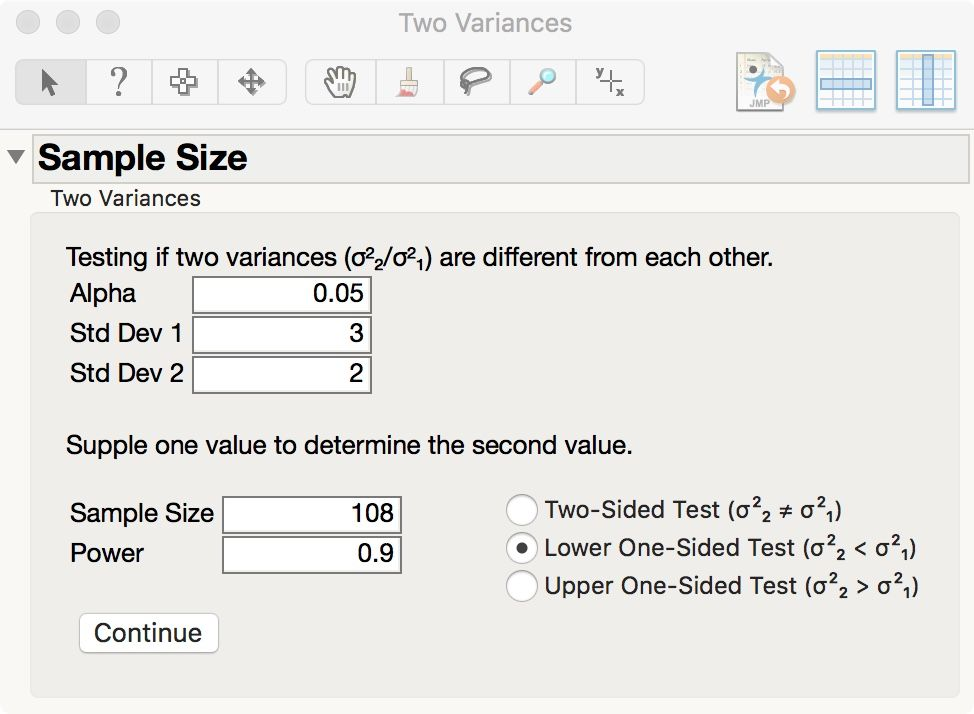Please note that I replaced the add-in after fixing a bug. If you have trouble estimating the power, then download the update and re-install. The current version is 1.4 (18Dec2018).

I just tried it and it worked without any problem. Are you logged in? Do you get an error message?

When you double click on the .jmpaddin JMP should open and prompt you to ask if you want to install the add-in.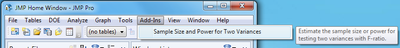If these steps don't help, post back and let me know where you're running into trouble and I'll try to assist further.

When I first tried this add-in, It worked, but it didn't make it into the add-ins menu.

Hi Mark,

Thank you for this tool. I am using JMP 13.1.0 and have encountered a few issues with the add-in.

1) The calculated sample size appears to be capped at NMT 302.
2) I found a table online to check the tool against, and I was getting different values for sample size at low power level. For example at alpha=.01, std1=1.0, std2=0.7, power=0.1, Lower One-Sided, the add-in gives me a sample size of 26.
But when I calculate this by hand, I get a sample size of 24.
var1/var2 = 1.0^2/0.7^2 = 2.0408
F^-1 of .99 with df of 11 and 11 (assuming total N=24) is .2241
F of (2.0408 * .2241) with same dfs is .8949
Power would be .1051

Did I make a mistake? Is your tool using a different formula?

1. I don't know what you mean by "NMT 302." There is no fixed upper limit to the sample size in this add-in.
2. My method is based on essentially the same approach as your method.
1. I don't know what on-line source you found for comparison so I cannot speak to its validity or its use in vetting this add-in.
2. The add-in iteratively increases the balanced sampling until the power exceeds the requested level.
3. I obtain power = 0.0949046952355616 for sample size 24 through manual computation. That level is less than the requested level = 0.1, so the add-in chose 26

This computation involves the tail of a distribution, so the numerical accuracy of the result requires careful attention to the precison of intermediate calculations or else round-off errors can propogate to the most significant digits.

1) see the first attached image. The sample size should be 1814 for this example. I entered in the Power and clicked "Continue" and 302 was generated in the Sample Size box.
2.1) The source is linked: NCSS document table and I have attached the table I used as the second image below. The first 4 values are different. I also cannot speak to its validity, so when I saw that it disagreed with the JMP tool I found an online guide for an excel tool to check the numbers, which I replicated. The table and the excel functions got the same results, which differ from the JMP tool.
2.2) That make sense, but I don't understand why I am seeing different numbers for the same inputs.
2.3) For sample size 24 I calculate a power of .10510478.

I put rounded values in the post above, but I use unrounded values in the calculation. I wonder if the excel function and the NCSS software use a a different number of digits from the JMP tool.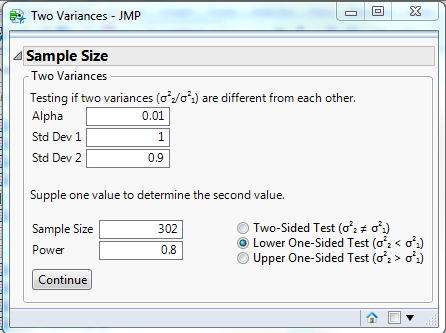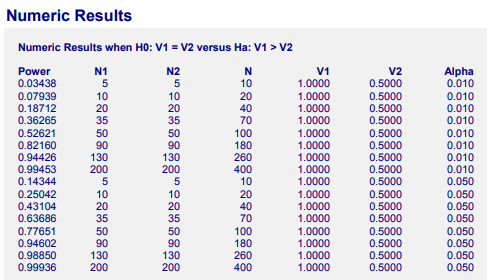1. I agree: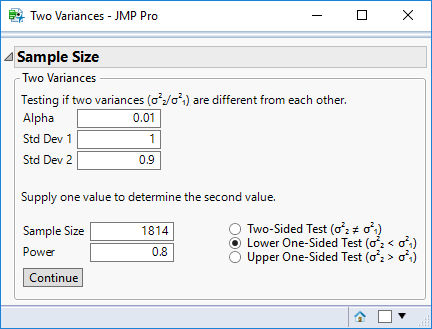2. It could be Excel. You can research published (peer reviewed journals) by  Dr. B.D. McCullough, Department of Decisions Sciences and Department of Economics, Drexel University, Philadelphia, PA. The built-in functions were found to be inaccurate, especially in the tails, even in recent versions. Excel was tested with a suite of vetted benchmarks for numerical accuracy of many statistical functions and methods.